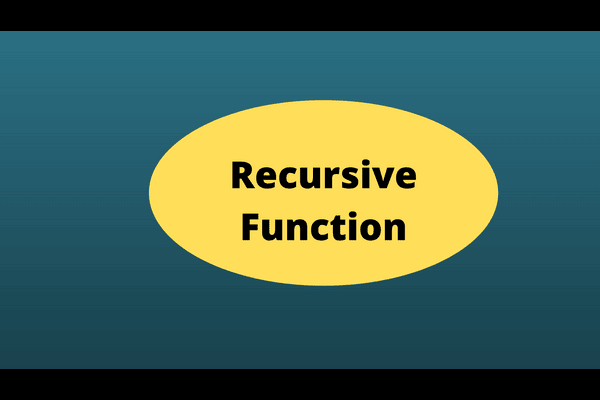Posts

Showing posts with the label Bash

Bash Recursive Function How to Write it QuicklyHere's a simple bash recursive function. That tells how to use it. You can write these in JavaScript and other scripting languages. This specific example shows you how to write it in Bash shell. Recursive in the shell script Calling the same function within it is called the Recursive function . How it works - we call itself and its contents.  Usually, the recursive functions go in the loop due to self-calling. Your code must have a condition that breaks the loop. Script logic #!/bin/bash for ((i = 1; i < 65; i++)) { ((arr[i - 1] = i)) } i = 1;  key = 8  function linear_search {  echo "Element value: \${arr[\$i-1]}"  if ((arr[i - 1] == key)) then  echo "Linear search found \$key on attempt \$i" return 0 ==> it breaks the loop  else ((i++)) linear_search == > Calling th same script fi  }  linear_search == > Calling the same script Output Here is the dissection of output. Displayed the array's elements until match-condition occurs. In the end, a displ